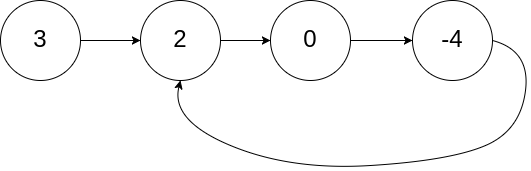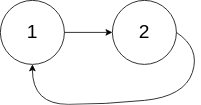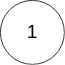## Problem Statement

Given a linked list, determine if it has a cycle in it.

To represent a cycle in the given linked list, we use an integer `pos` which represents the position (0-indexed) in the linked list where tail connects to. If `pos` is `-1`, then there is no cycle in the linked list.

Example 1:

```Input: head = [3,2,0,-4], pos = 1
Output: true
Explanation: There is a cycle in the linked list, where tail connects to the second node.
```Example 2:

```Input: head = [1,2], pos = 0
Output: true
Explanation: There is a cycle in the linked list, where tail connects to the first node.
```Example 3:

```Input: head = , pos = -1
Output: false
Explanation: There is no cycle in the linked list.
```